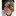## floating point math limit?

Hi All,

Is there some odd limit to the amount of floating point stuff you can do in a program?? The reason I ask is that I have been working on the following program which I couldn't get to work and have been slowly stripping out code to see what the problem is. It runs fine when I take the marked section out but won't work with it in - even though I have modified it so that the section os code doesn't do anything other than crunch some floating point numbers???

Can anyone help me out?

#include "mbed.h"
#include "AX12.h"

struct LegAngles {
float Femer;
float Tibia;
};

LegAngles GetAngles(int X, int Y) {

/* IF I TAKE THIS BOLD SECTION OUT THE PROGRAM RUNS OK... */
float Hypotenuse, S, C, Area, Opposite, Alpha, Beta, Gamma, Adj = Y, Opp = X;
Hypotenuse = (X*X)+(Y*Y);
Hypotenuse = sqrt(Hypotenuse);
C = Hypotenuse;
S = (100 + 100 + C)/2;          // Calculate the semi-perimeter
Area = S*(S-100)*(S-100)*(S-C);
Area = sqrt(Area);
Opposite = (2*Area)/Hypotenuse;
Alpha = Opposite / 100;
Alpha = asin(Alpha);
Beta = Opposite / 100;
Beta = asin(Beta);
Gamma = atan(Gamma);

LegAngles Angle;

Angle.Femer = X + 150;
Angle.Tibia = Y;
return Angle;
}

int main() {
AX12 myax12_Hip (p9, p10, 1), myax12_Knee (p9, p10, 2);

Timer t;
int x, y;

t.start();
while(1) {
t.reset();

x = (50 * t.read()) - 50;
y = 180;
}
else{
x = (50 * (4 - t.read())) - 50;
y = 200;
}

}
}

Define "won't work". Doesn't compile, doesn't run, produces bad results?

P.S. please use `<<code>>..<</code>>` tags to mark source code fragments.

Sorry, I should have been clearer. When the

<<code>>

float Hypotenuse, S, C, Area, Opposite, Alpha, Beta, Gamma, Adj = Y, Opp = X;

Hypotenuse = (X*X)+(Y*Y);
Hypotenuse = sqrt(Hypotenuse);
C = Hypotenuse;
S = (100 + 100 + C)/2;          // Calculate the semi-perimeter
Area = S*(S-100)*(S-100)*(S-C);
Area = sqrt(Area);
Opposite = (2*Area)/Hypotenuse;
Alpha = Opposite / 100;
Alpha = asin(Alpha);
Beta = Opposite / 100;
Beta = asin(Beta);
Gamma = atan(Gamma);

<</code>>

is put in the 2 AX12 servos i'm trying to control don't move and when I put in a printf statement to see what is going on the program appears to hang at the point they were addressed. The program complies fine with no errors or warnings.

I can second this. I had experimented yesterday with PWM output, and have seen the same behavior. My program was even much simpler, and the culprit seemed to be a printf-statement printing two floats. The behavior was that the PWM output stayed with the first written value, and was never changed.

Hi Martin, Hendrick,

Thanks for reporting this. I've been investigating, and have been able to reproduce the PwmOut problem (thanks for the program!). Whilst I haven't ruled it out yet, I don't think the problem is actually related to the mbed libraries or PwmOut. It is looking to me more like something to do with the underlying compiler/compiler libraries or our interaction with them.

Martin, I don't have a AX12 to hand; would it be possible to try and provide me an example that exhibits the behaviour, without relying on the AX12's being connected? I'd like to investigate both reports to help track this down and ensure it solves both cases.

Thanks! Simon

Hi again,

I think we've got to the bottom of this and now have a fix. We're going to do some more testing, but we'll aim to get an update to the compiler asap.

Thanks again for the report and examples.

Simon

Please post the details when you confirm it. I'm curious at how such innocent-looking code could break things.

####Simon Ford wrote:

I think we've got to the bottom of this and now have a fix. We're going to do some more testing, but we'll aim to get an update to the compiler asap.

Any forecast of when the compiler update will be ready?

In the meantime, could you give us an idea of how the problem is triggered? I'm debugging an obscure problem, and can't help wondering if I've run into this library problem.

-hb

Hi Hexley, All,

We've just applied a patch to the compiler which should now fix this bug, as shown in your PwmTest example. FYI, it appears to have been caused by a corner-case optimisation bug, fixed in this latest release.

I've tested it with your example and all passes, but please report back if you have further problems.

Thanks again, Simon